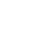# Sources of Energy MCQ Objective questions

Sources of Energy MCQ Objective questions Class 10 Science Chapter 14 NCERT

#### 1. Energy remains conserved during a physical and chemical reaction. Then why we still keep on finding new sources of energy?

• a. Though energy is conserved, it is not always convertible.
• b. A chemical reaction reduces energy output.
• c. The storage of energy is not economical.
• d. None.
1. a

#### 2. A hydroelectric dam use _ _ _ _ energy of stored water to generate electrical power.

• a. Kinetic energy
• b. Nuclear energy.
• c. Potential energy.
• d. None.
2. c

#### 3. When we burn a candle, we use _ _ _ _  energy of wax to get light and heat energy.

• a. Electrical energy.
• b. Chemical energy.
• c. Potential energy.
• d. Kinetic energy.
3.b

#### 4. A reaction is exothermic when we get _ _ _ _ _ energy from the reaction.

• a. Heat energy.
• b. Chemical energy.
• c. Electrical energy.
• d. Kinetic energy.
4.a

• a. Yes.
• b. No.
5.b

#### 6. Where the energy of a candle go afterburning?

• a. Dissipated in the environment.
• b. Converted into the mass.
• c. Converted into heat.
• d. A and C both as heat and light energy of a candle, later goes to the environment.
6.d

• a. 0ºK
• b. 0ºF
• c. 273ºK
• d. None.
7.c

#### 8. Formula for converting ºC  to ºK is:

• a. ºK = ºC + 275ºC
• b. ºK = ºC + 273ºC
• c.  ºK = ºC
• d. ºK = ºC – 273ºC
8.b

#### 9. Formula for converting ºC  to ºF is:

• a. 9/5 ºC  = ºF – 32
• b. ºC  = ºF
• c. ºF = ºC + 275ºC
• d. none.
9.a

• a. -198ºC
• b. 0ºC
• c. -273ºC
• d. None.
10.c

#### a. Cheap.11. A good fuel is:

• b. Easily available.
• c. Clean.
• d. All.
11.d

#### 12. India depends mostly on _ _ _ _ to meet its energy demand.

• a. Coal.
• b. Hydroelectric dam.
• c. Nuclear power.
• d. Petroleum and natural gas.
12.a

#### 13. Clean fuel means a fuel that:

• a. look tidy.
• b.can be filtered off.
• c. is non-polluting.
• d. None.
13.c

#### 14. A thermoelectric plant converts _ _ _ _ _ energy of coal into electrical energy.

• a. Chemical.
• b. Potential.
• c. Heat.
• d. None.
14.a

#### 15. Following is an example of biomass fuel.

• a. broken twigs and branches of a tree.
• b. Cow dung.
• c. Crop residue.
• d. All.
15.d

#### 16. Bio-mass is a _ _ _ _ fuel while biogas produced from it is a _ _ _ _ fuel.

• a. Polluting, clean.
• b. Highly efficient, non-efficient.
• c. Low efficient, High efficient
• d. a and c both.
16.a

#### 17. Following is/are examples of renewable energy:

• a. Wind energy.
• b. Hydro energy.
• c. Solar energy.
• d. All of the above.
17.d

#### 18. Country of winds is :

• a. Netherland.
• b. Denmark.
• c. Cambodia.
• d. Switzerland.
18.b

#### 19. Why we consider solar energy as a renewable source of energy.

• a. Sun will provide energy for billions of years from now.
• b. Sun is a permanent celestial body.
• c. It is not considered renewable.
• d. None.
19.a

#### 20. Ocean thermal energy use the following principle::

• a. There is a temperature difference between the surface of ocean water and deep water.
• b. The heat produced from the volcano situated under the sea.
• c. The thermal energy of ocean water.
• d. None.
20.a

How do Organisms Reproduce MCQ.

 1. a 9.a 17.d 2. c 10.c 18.b 3.b 11.d 19.a 4.a 12.a 20.a 5.b 13.c 6.d 14.a 7.c 15.d 8.b 16.a

Sources of Energy MCQ Objective questions Class 10 Science Chapter 14 NCERT

Ref: Chapter 14, NCERT.

### 2 thoughts on “Sources of Energy MCQ Objective questions”

1.It is too useful for me. My chemistry teacher Mr. Shyam Sir given me and my classmate this link on our school group and it is too helpful for me and my friends. This is the time of pandemic and in this time it becomes too useful for me and my friends.
Thanks to my Sir and to this website.

1.yes me too